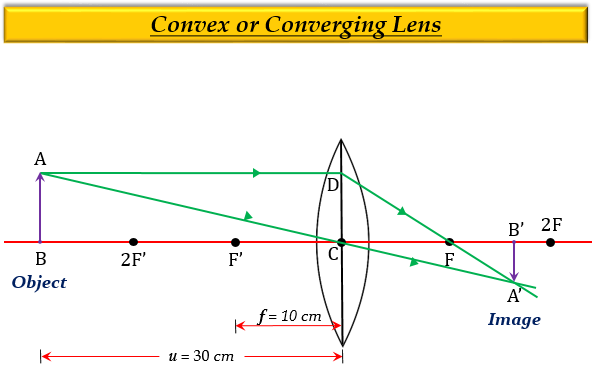# One-half of a convex lens is covered with a black paper. Will this lens produce a complete image of the object? Verify your answer experimentally. Explain your observations.

When one-half of a convex lens is covered with black paper. Yes, such a lens can produce an image of a complete object placed at a distance from the lens. Because the light coming from the object can be refracted from the other half of the lens. The image formed will be real, inverted, and diminished.

The ray diagram for the justification of the answer is as follows:Let,

Object size, $h=+4cm$

Object distance, $u=–15cm$

Focal length, $f=+20cm$

To find: Image distance, or position $v$, nature and size of the image, $h'$.

Solution:

From the lens formula, we know that-

$\frac {1}{f}=\frac {1}{v}-\frac {1}{u}$

Substituting the required values, we get-

$\frac {1}{20}=\frac {1}{v}-\frac {1}{(-15)}$

$\frac {1}{20}=\frac {1}{v}+\frac {1}{15}$

$\frac {1}{v}=\frac {1}{20}-\frac {1}{15}$

$\frac {1}{v}=\frac {3-4}{60}$

$\frac {1}{v}=-\frac {1}{60}$

$v=-60cm$

Thus the screen image distance or position, $v$ is 60 cm from the lens, and the minus sign implies that the image forms in front of the lens (on the left side). Also, the image will be virtual as it forms on the left side of the lens.

Now,

From the magnification formula, we know that-

$m=\frac {v}{u}=\frac {h'}{h}$

Substituting the required values, we get-

$\frac {-60}{-15}=\frac {h'}{4}$

$4=\frac {h'}{4}$

$h'=+16cm$

Thus, the height or size of the image, $h'$ is 16 cm, and the plus sign implies that the image forms erect (upright ).

Therefore, the image formed will be virtual, erect, and magnified.

Updated on: 10-Oct-2022

175 Views